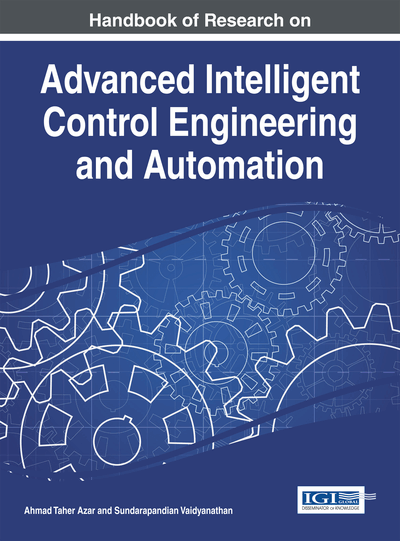# On Proportional Plus Derivative State Feedback H2 Control for Descriptor Systems

Rim Zaghdoud (National School of Engineering of Tunis, Tunisia), Salah Salhi (National School of Engineering of Tunis, Tunisia) and Moufida Ksouri (National School of Engineering of Tunis, Tunisia)
DOI: 10.4018/978-1-4666-7248-2.ch005

## Abstract

This chapter studies the control problem for linear singular systems. Firstly a proportional plus derivative feedback controller for continuous and discrete cases for descriptor systems is given, satisfying the closed loop systems normal and stable states. A necessary and sufficient condition for the solvability of this problem should be obtained in terms of linear matrix inequality. In second part, the designed controller is required to yield a cost function with guaranteed upper bound for the continuous and discrete case and also an extension for robust control is developed. Numerical examples are included to show the effectiveness of the proposed result.
Chapter Preview
Top

## 1. Introduction

The singular systems have attracted the attention of a lot of researchers due to their capacity to preserve the structure of physical systems and also encompass impulsive behaviors and non-dynamic constraints. There has been a growing interest in the control of descriptor systems since such systems have extensive applications in economic systems, large scale systems and other areas (Dai, 1989; Lewis, 1986). Many fundamental concepts and results based on the theory of ordinary systems have been successfully developed to descriptor systems. Let’s us quote the notion of observability, controllability, stability and stabilization technique (Dai, 1989; Xu & Lam, 2006) as well as results including robustness aspects (Xu, & Lam, 2006; Chaabane et al., 2011; Sari et al., 2008; Ren & Zhang, 2010; Faria et al., 2009; Bara, 2011).

Since the stability of dynamic system is well regarded as one of the most important properties of systems, much attention has been focused on the problems of robust stability and robust stabilization of descriptor systems. It is known that the robust stability problem for singular systems is much more complicated than that for ordinary systems because it requires to consider not only stability but also absence of impulses and regularity simultaneously (Fang & Chang, 1993; Fang & Chang, 1994; Lin et al., 1997; Xu & Lam, 2003; Xu, 2002) while the latter two do not arise in the conventional state space context. This justifies the two approaches used in relevant literature to resolve the stability problem: one is to develop the admissibility concept which requires verifying the stability, regularity and non-impulsiveness of the descriptor system. The other is limited to ensure the stability and the regularity of the system by the design of controller which make the system be normal or in other words the non-singularity of matrix E. In Xu et al. (2002) an algebraic approach was proposed to solve the robust stabilization problem via a linear matrix inequality (LMI), as well as to singular systems with time delays was developed. For the generalized quadratic stabilization, necessary and sufficient conditions were given in terms of Riccati inequality in Lin et al. (2000). The robust stabilization problem for uncertain discrete time singular systems was investigated in Xu et al. (2001), where a sufficient condition for generalized quadratic stabilization was developed. Under some assumptions about the system matrices, this sufficient condition was modified to be necessary. In Xu and Lam (2004), the robust stabilization problem is solved through state feedback controller where the parameters uncertainties appearing in both the state and input matrices and the concepts of generalized quadratic stability and stabilization are introduced. In the same paper, a necessary and sufficient condition is given in terms of a strict LMI such the system will be admissible. Again, the robust stabilization problem through state feedback for uncertain singular systems is given in the work of Xu et al. (2002). In Xu and Yang (1999), to reduce the conservatism of the stabilization quadratic, a PDL approach is used to salve the robust static output feedback admissibility problem for the descriptor systems case. However, in practical control system design, the robust performance is also required as well as robust stability against the uncertainty of the plant model (Boukas, 2005; Boukas et al., 2003; Dragan, 2005; Dragan, et al., 1999; Mahmoud and Shi, 2003; Shi, 1999; Xia & Jia, 2003a; Xia & Jia, 2003b). Bothandof the system have been widely used to bring clarity to the system performance in past studies and the problems of robustandcontrol for systems with uncertain parameters have been extensively studied in the past decade (Ikeda et al., 2000; Masubuchi, 1997; Takaba, 1998). In More recent times, much work has been done on multiobjective optimization problems and specially, the mixedcontrol problem has received considerable attention (Bernstein & Haddad, 1981; Doyle et al., 1994; Geromel et al., 1992; Khargonekar & Rotea, 1991; Rotea & Khargonekar, 1991; Zhou et al., 1994). In work Xu and Lam (2004), necessary conditions were given to minimize an upper bound on theperformance under aconstraint.

## Key Terms in this Chapter

H2 Control Problem: Consists of finding a controller which stabilizes the system and which minimizes the cost function where is the norm.

Singular Systems: A dynamic systems that the matrix on the derivative of the state, that is E in (5), is generally singular.

Stabilization: Is concerned with the designing a controller of a prescribed type such that the closed loop system is stable.

PD State Feedback Controller: Is designed for the closed loop system to be normal and stable.

Robust Control: Considers the design of decision or control rules that fare well across a range of alternative models. Thus robust control is inherently about model uncertainty, particularly focusing on the implications of model uncertainty for decisions.

Normalization: Is concerned with designing a controller for a singular system such that the closed loop system is normal.

LMIs: A linear matrix inequality is a convex constraint. They are a useful tool for solving a wide variety of optimization and control problems.

## Complete Chapter List

Search this Book:
Reset